Processing ......FreeComputerBooks.com Links to Free Computer, Mathematics, Technical Books all over the World

R for Data Science: Visualize, Model, Transform, Tidy, and Import Data
Want to measure length or width of any objects on the earth? Try GIS Visualizer.
• Title: R for Data Science: Visualize, Model, Transform, Tidy, and Import Data
• Author(s) Hadley Wickham (Author), Garrett Grolemund (Author)
• Publisher: O'Reilly Media; 1 edition (2016); eBook (Creative Commons Licensed)
• License(s): CC BY-NC-ND 3.0 US
• Hardcover/Paperback: 518 pages
• eBook: HTML
• Language: English
• ISBN-10/ASIN: 1491910399
• ISBN-13: 978-1491910399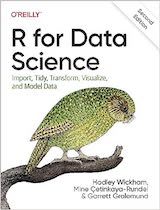Book Description

This book will teach you how to do data science with R: You'll learn how to get your data into R, get it into the most useful structure, transform it, visualize it and model it.

What exactly is data science? With this book, you'll gain a clear understanding of this discipline for discovering natural laws in the structure of data. Along the way, you’ll learn how to use the versatile R programming language for data analysis.

Whenever you measure the same thing twice, you get two results—as long as you measure precisely enough. This phenomenon creates uncertainty and opportunity. Author Garrett Grolemund, Master Instructor at RStudio, shows you how data science can help you work with the uncertainty and capture the opportunities. You'll learn about:

• Data Wrangling - how to manipulate datasets to reveal new information
• Data Visualization - how to create graphs and other visualizations
• Exploratory Data Analysis - how to find evidence of relationships in your measurements
• Modelling - how to derive insights and predictions from your data
• Inference - how to avoid being fooled by data analyses that cannot provide foolproof results

Through the course of the book, you'll also learn about the statistical worldview, a way of seeing the world that permits understanding in the face of uncertainty, and simplicity in the face of complexity.

• Hadley Wickham is Chief Scientist at RStudio and a member of the R Foundation. He builds tools (both computational and cognitive) that make data science easier, faster, and more fun. His work includes packages for data science (ggplot2, dplyr, tidyr), data ingest (readr, readxl, haven), and principled software development (roxygen2, testthat, devtools). He is also a writer, educator, and frequent speaker promoting the use of R for data science. Learn more on his homepage, http://hadley.nz.
Reviews, Rating, and Recommendations: Related Book Categories: Read and Download Links:Similar Books:
•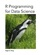R Programming for Data Science (Roger D. Peng)

This book is about the fundamentals of R programming. Get started with the basics of the language, learn how to manipulate datasets, how to write functions, and how to debug and optimize code. You will have a solid foundation on data science toolbox.

•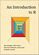An Introduction to R (Alex Douglas, et al.)

The main aim of this book is to help you climb the initial learning curve and provide you with the basic skills and experience (and confidence!) to enable you to further your experience in using R.

•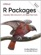R Packages: Organize, Test, Document, and Share Your Code

Turn your R code into packages that others can easily download and use. This practical book shows you how to bundle reusable R functions, sample data, and documentation together by applying author's package development philosophy.

•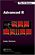Advanced R Programming (Hadley Wickham)

This book presents useful tools and techniques for attacking many types of R programming problems. It not only helps current R users become R programmers but also shows existing programmers what's special about R.

•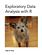Exploratory Data Analysis with R (Roger D. Peng)

This book covers the essential exploratory techniques for summarizing data with R. These techniques are typically applied before formal modeling commences and can help inform the development of more complex statistical models.

•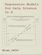Regression Models for Data Science in R (Brian Caffo)

The book gives a rigorous treatment of the elementary concepts of regression models from a practical perspective. The ideal reader for this book will be quantitatively literate and has a basic understanding of statistical concepts and R programming.

•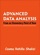Advanced Data Analysis using R (Cosma R. Shalizi)

This is a textbook on data analysis methods, intended for advance undergraduate students who have already taken classes in probability, mathematical statistics, and linear regression. All examples implemented using R.

•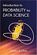Introduction to Probability for Data Science (Stanley Chan)

This book is an introductory textbook in undergraduate probability in the context of data science to emphasize the inseparability between data (computing) and probability (theory) in our time, with examples in both MATLAB and Python.

•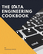Data Engineering Cookbook: The Plumbing of Data Science

This is a practical and comprehensive guide. You will learn the basics of data engineering. Then you will learn the technologies and frameworks required to build data pipelines to work with large datasets.

•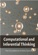Computational and Inferential: The Foundations of Data Science

Step by step, you'll learn how to leverage algorithmic thinking and the power of code, gain intuition about the power and limitations of current machine learning methods, and effectively apply them to real business problems.

•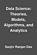Data Science: Theories, Models, Algorithms, and Analytics

It provides a bucket full of information regarding Data Science, covers a wide variety of sections by giving access to theories, data science algorithms, tools and analytics. You'll explore the right approach to best practices to guide you along the way.

•The Data Science Handbook: Advice and Insights

This book covers the essential exploratory techniques for summarizing data with R. These techniques are typically applied before formal modeling commences and can help inform the development of more complex statistical models.

•Python Data Science Handbook: Essential Tools (Jake VanderPlas)

Several resources exist for individual pieces of this data science stack, but only with the Python Data Science Handbook do you get them all - IPython, NumPy, Pandas, Matplotlib, Scikit-Learn, and other related tools.

Book Categories
 :All CategoriesRecent BooksMiscellaneous BooksComputer LanguagesComputer ScienceData Science/DatabasesElectrical EngineeringJava and Java EE (J2EE)Linux and UnixMathematicsMicrosoft and .NETMobile ComputingNetworking and CommunicationsSoftware EngineeringSpecial TopicsWeb Programming
Other Categories